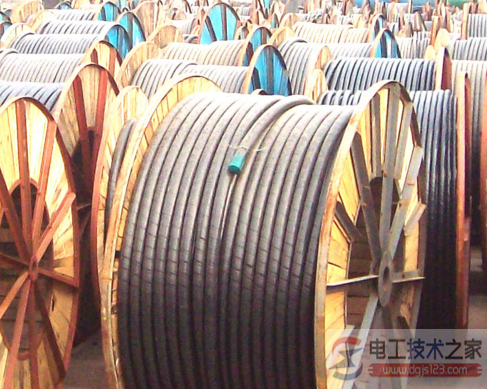`>`首页 > 电工基础 > 电工材料`>`电缆损耗计算公式 正文

# 电线电缆材料用量及电缆损耗计算公式

###### 来源：电工天下 编辑：小电工发布时间：2016-05-28 09:02:131、导体用量：(Kg/Km)=d^2 * 0.7854 * G * N * K1 * K2 * C / d=铜线径 G=铜比重 N=条数 K1=铜线绞入率 K2=芯线绞入率 C=绝缘芯线根数

2、绝缘用量：(Kg/Km)=(D^2 - d^2)* 0.7854 * G * C * K2 D=绝缘外径 d=导体外径 G=绝缘比重 K2=芯线绞入率 C=绝缘芯线根数

3、外被用量：(Kg/Km)= ( D1^2 - D^2 ) * 0.7854 * G D1=完成外径 D=上过程外径 G=绝缘比重

4、包带用量：(Kg/Km)= D^2 * 0.7854 * t * G * Z D=上过程外径 t=包带厚度 G=包带比重 Z=重叠率(1/4Lap = 1.25)

5、缠绕用量：(Kg/Km)= d^2 * 0.7854 * G * N * Z d=铜线径 N=条数 G=比重 Z=绞入率

6、编织用量：(Kg/Km)= d^2 * 0.7854 * T * N * G / cosθ θ = atan( 2 * 3.1416 * ( D + d * 2 )) * 目数 / 25.4 / T d=编织铜线径 T=锭数 N=每锭条数 G=铜比重

1.护套厚度：挤前外径×0.035+1(符合电力电缆,单芯电缆护套的标称厚度应不小于1.4mm，多芯电缆的标称厚度应不小于1.8mm)

2.在线测量护套厚度：护套厚度＝(挤护套后的周长—挤护套前的周长)/2π 或护套厚度＝(挤护套后的周长—挤护套前的周长)×0.1592

3.绝缘厚度最薄点：标称值×90%-0.1

4.单芯护套最薄点：标称值×85%-0.1

5.多芯护套最薄点：标称值×80%-0.2

6.钢丝铠装：根数= ｛π×(内护套外径+钢丝直径)｝÷(钢丝直径×λ) 重量=π×钢丝直径2×ρ×L×根数×λ

7.绝缘及护套的重量=π×(挤前外径+厚度)×厚度×L×ρ

8.钢带的重量=｛π×(绕包前的外径+2×厚度-1) ×2×厚度×ρ×L｝/(1+K) 9.包带的重量=｛π×(绕包前的外径+层数×厚度)×层数×厚度×ρ×L｝/(1±K) 其中:K为重叠率或间隙率，如为重叠，则是1-K；如为间隙，则是1+K ρ为材料比重；L为电缆长度；λ绞入系数。

(3.14/4)*单线直径*单线直径*单线根数*8.9*1.012*芯数*1.008=...千克/千米

`相关文章`